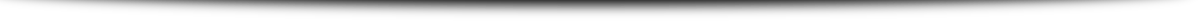# Create A Graph – A Picture Is Worth A Thousand Numbers

You have data!  The problem is to pull meaning out of it.  The data has no value if you can’t understand it.

The solution is to visualize that data.  One of the simplest ways to do just that is with graphs.  Graphs have a way of letting you see the big picture that is hidden within the mass of numbers.

Types Of Graphs

There are several types of graphs.  Each has its strengths and weaknesses.  The following list shows the more common graphs with their pros and cons:

Pros                                    Cons

Line Graphs –     Great for seeing trends and seasonality in data.  –  Not good with small amounts of data.

Pie Graphs –     Good for showing the percentage of the whole.  –   One trick pony! No other uses.

Bar Graphs –     Better with small amounts of data.      –    Not good with large amounts of data.

Uses For Graphs

Graphs have an amazingly wide number of uses.  Some of these are listed below:

Show trend over time.

Illustrates data seasonality.

A visual indicator of volatility.

A predictor of future results.

What portion each part is of the whole.

A means of making comparisons between multiple sets of data.

Shows when a preset standard is being met.

Provides a starting point for regression analysis.

Initial analysis for curve fitting.

Helps visualize the relationship between two or more variables.

Simplifies reporting.

Identifies opportunities.

The Language Of Graphs

Graphs have a set of vocabulary that is necessary to know to fully understand their meaning.  Most of the terms used come right outof your algebra textbook.   If you know algebra, then you probably already know theses terms.  If you don’t know algebra then you needto learn these terms and their definitions.

X –  It is common practice to call the horizontal  x values.

Y – The vertical values are referred to as the y values.

X-axis – Separates the y positive values from the y negative values.

Y-axis – This is the vertical line which separates the x positive values from the x negative values.

Slope – This is simply the slant of the graph.  A positive value says the graph is rising.  A negative value says the graph is falling.

Variables – These are the two part values consisting of a dependent variable and an independent variable.  An example might be  a graph of monthly expenses.  The x variable is the month and the y variable is the expense.  A data point might be  February for \$3000.  When March comes along, the value might be \$2500.

How To Create A Graph

It used to be necessary to have graph paper to create a graph.  Now we have computers.  Spreadsheets, specifically Excel, are often used to create computer graphs.  Online resources are available to create your graph and even print it on your own printer.  These resources are shown below.

Summary

When you create a graph you have made your data visual.  When it is visual, it is much more comprehensible to the human mind.  You have transformed your data into something that communicates meaning more clearly.

Posted in:
Articles by:
Published: